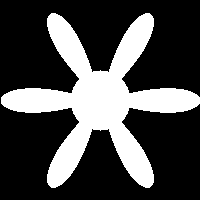HTMSTM(a)A / People / Matt Keeter / Tutorials

# Behind the Curtain

## What's going on here?

After the first tutorial, you might be wondering how this all works. What kind of geometry engine is allowing you to create, transform, and combine shapes into complex designs?

Surprisingly, the system has very little knowledge of geometry. Instead, it operates on arbitrary strings of math and logic.

Let's rewrite the first example without the build-in library:

 `````` cad.xmin = -1 cad.xmax = 1 cad.ymin = -1 cad.ymax = 1 cad.function = 'X*X + Y*Y < 1' ``````Behind the scenes, a powerful solver evaluates the mathematical expression for every pixel in the image. If the pixel is true, then that pixel is colored white; otherwise, it is left black.

## Transformations

All of the shapes, transformations, and combinations are implemented as operations on these mathematical expressions. For example, let's look at a simple implementation of `move(part, dx, dy, dz)`

``````def move(part, dx, dy, dz = 0):
part = part.replace('X','(X-%f)' % dx)
part = part.replace('Y','(Y-%f)' % dy)
part = part.replace('Z','(Z-%f)' % dz)
return part
``````

This code is doing string substitutions on the original representation string. Every instance of `X` is replaced with `(X - dx)`, where `dx` is the amount that we want to move along the x axis; similarly for y and z movement.

By chaining these operations together, we can build long, complex math expressions that represent large, complex shapes.

## Example

Here is an example that uses raw math expressions to generate a six-petaled flower.

 `````` cad.xmin = -1 cad.xmax = 1 cad.ymin = -1 cad.ymax = 1 cad.function = '(X*X + Y*Y) < cos(atan2(Y, X)*6)' ``````Of course, these expressions can be combined with the standard library of shapes as expected.

 `````` from cad_shapes import * cad.xmin = -1 cad.xmax = 1 cad.ymin = -1 cad.ymax = 1 petals = '(X*X + Y*Y) < cos(atan2(Y, X)*6)' cad.function = add(petals, circle(0, 0, 0.3)) ``````If you get nervous when trigonometry starts showing its ugly face, don't panic! Understanding the underpinnings gives you more power to define your own shapes and transformations, but it's not mandatory.

It's completely possible to design with the standard library of shapes, without ever touching the math expressions at the heart of the design format.